## Monday, April 27, 2009

### The Dot and Cross Products

The dot product and cross product of two vectors are tools which are heavily used in physics. As such, they are typically introduced at the beginning of first semester physics courses, just after vector addition, subtraction, etc. Although they are not strictly required for these intro courses (see , for example), they make the development and computations of work and energy, torque, and electromagnetism far simpler.

Unfortunately, they are consistently introduced in an awful way: by straight definition. That is, using the dot product for example, for two vectors and they say something like
We define the dot product between and as:
or,
where is the angle between them.
Then, for the cross product, either they use an equation like the latter of the above two equations coupled with the right-hand rule,'' or a strange algebraic combination of the components of and , often simplified'' with help of a startling determinant.1 See , , ,  and  as a few examples. Although a few of these give a geometric interpretation after the fact, it is usually in passing, and does not really contribute to their discussion. These approaches are not limited to textbooks, either. See  for an in-class lecture example.

In these examples, the dot product is introduced first and then the cross product. From one standpoint this makes some sense -- the dot product is definitionally simpler and usually easier to calculate. However, from a conceptual standpoint, I think this order is backwards. Furthermore, in my experience, students, by and large, miss the physical and graphical significance of these definitions, and upon encountering the concepts of work or torque later on, take the resulting expressions purely as definitions as well.2 This is yet another example of the fact that definition explanation.

Personally, it is my inclination to wait to introduce these products until they're needed, thus motivating the discussion in the first place. However, I do understand the notion of getting it over with,'' and, it's possible that introducing them as abstract concepts lends to easier application of the concepts to general problems. In any case, my discussion follows the latter approach (for better insertion into standard texts) and presupposes understanding of vector basics: addition, decomposition, etc..

A better way
The Cross Product
(a) Geometrical view of the cross product as the parallelogram area.

(b) Graphical derivation of area for two 2D arbitrary vectors, from .
Figure 1: The 2D cross product of vectors and .

Say we have two vectors and with lengths and , and we want to find something which is a measure of how much of is perpendicular to . Looking at Fig. 1(a), we can see that the area of the parallelogram sided by the two vectors is such a measure. The area of a parallelogram is
(1)
which, for our case, is the same as
(2)
That is, you can only have an area if you have a base'' and a height'' perpendicular to the base. Thus area is a good measure of perpendicularity.3

There are two different ways of calculating this area. If the angle between the two vectors is , as in Fig. 1(a), we see that, choosing as the base'' we can write the height'' as . Alternatively, choosing as the base, we write the perpendicular part as . Then the area is
.
(3)
However, if we don't know angle between them, we're not completely out of luck. If you look at Fig. 1(b), you can see that for a simple, two-dimensional case, we can express the area in terms of the and components of and :
(4a)
Of course, I could just have easily labeled the axes and which would give a different area
(4b)
or and , which would give yet another area
(4c)

If all we've done is relabel our axes, keeping and fixed, then we wouldn't expect the size of these areas to be different -- and they're not. However, although the amount of area is the same, in a way the areas are different in that they're facing different directions in each case. So, we need a way to distinguish these three areas from each other, and from an arbitrarily oriented area. What we'll do is pick a vector perpendicular to both to -- and thus perpendicular to the area of the parallelogram -- with magnitude equal to the area. We'll call this vector
(5)
and say it's the result of a cross product'' of and . However, in principle, we have a choice of two such perpendicular vectors. In Fig. 1, for example, we could choose the vector pointing in either the or direction. Additionally, this arbitrariness can be seen in choosing whether to measure the angle in (3) from to or vise-versa.

So, as a matter of convention, we'll decide to always measure angles from the first term in the cross product ( in (5)) such that
(6)
so if the fingers in your right hand point along the little arcs we draw for angles, your thumb points in the direction that this vector goes. Thus,
(7)
since your hand would curl in the other direction. This is called the Right-Hand Rule.'' Then, the areas we discussed in equations (4) become
(8a)
(8b)
and
(8c)
where the subscripts tell us which coordinate plane the two crossed vectors are in. Thus, the cross product represents how much these two vectors point in perpendicular directions, and is a signed area vector perpendicular to the plane described by and .

(a) Geometrical view of the 3D cross product as the parallelogram area.
(b) Looking at the area from the xy-plane (dashed outline), the yz-plane (shaded) and the zx-plane (solid).
Figure 2: The 3D cross product of vectors and and the decomposed area.

So far, though, we've only discussed vectors which have only two coplanar components. But it's fairly straightforward to generalize to arbitrary 3D vectors. See Fig. 2(a), for example. Here the area vector, and hence the cross product vector, is pointing in a complicated direction. However, we know we can decompose any vector into its , and components, and this area vector is no different:
(9)

All we need to do is find out how much area is pointing in each direction. To do that, look at Fig. 2(b). This picture shows what the area between the two vectors looks like if we look only at two coplanar components at a time -- in other words the , and components of the area. But we already know what each of these areas are from (8)! So, then we can combine these equations and write the cross product
(10)

The Dot Product

Having discussed the perpendicularity of two vectors, it's natural to ask if there's a similar measure of the parallelity of two vectors. There are two ways of doing this. The way I'll do it first is explicitly geometrical, the second way is only implicitly geometrical.

Say we have two vectors and again, and we want to know how much of is pointing (projected) along . From Fig. 3 we see that this is equal to
(11)
Similarly, the amount of that is projected along is
(12)
Now, it would be nice if we could have one statement which somehow combined the these two statements and gave a measure both of how much of is pointing along and of how much of is pointing along ; that is, a measure of how much these two vectors point in the same direction. Additionally, since (2) used a multiplicative combination of the two vectors as a measure of perpendicularity, we'll try a similar multiplicative measure here, as well.

If we multiply (11) by and (12) by we can write a single, symmetric statement
(13)
and say it's the result of a dot product'' of and , which amounts to multiplying together the parallel parts of two vectors. Here, too, if we don't know the angle between them, we're not out of luck. For a vector written in component form, it's straightforward to multiply the parallel parts together:
(14)

However, unlike the cross product which gave us an actual area with a natural direction, this area-like structure is actually a measure of non-area'' and doesn't really have a natural direction. Although we could, completely arbitrarily, define a direction for this dot product,4 and thus make it a vector as well, to the best of my knowledge such a quantity does not have any uses in physics, so we'll leave it alone and treat it only as a number (scalar).

Alternatively, we know that the largest area possible between two vectors occurs when they are perpendicular to each other, where the area is (you can also see this from (3)). If we are interested in the maximal amount perpendicular'' we can write
(15)
where they are squared to take care of sign problems. Now, when they are completely parallel there is no area, and we're left only with non-area, which, also, can't be larger than the total maximum area, so
(16)
as well.

Then using a rough analogue to the Pythagorean theorem we see that
(17)
which, choosing the positive root, is the same as (13).

References
 F.W. Sears and M.Z. Zemansky. University Physics. Addison-Wesley, Reading, MA, 2nd edition, 1955.
 D. Halliday amd R. Resnick and J. Walker. Fundamentals of Physics. John Wiley & Sons, Inc., 7 edition, 2005.
 G.R. Fowles and G.L. Cassiday. Analytical Mechanics. Thomson Brooks/Cole, Belmont, CA, 7th edition, 2005.
 J.R. Reitz, F.J. Milford, and R.W. Christy. Foundations of Electromagnetic Theory. Addison-Wesley, 4th edition, 1992.
 D.J. Griffths. Introduction to Quantum Mechanics. Pearson Prentice Hall, 2nd edition, 2005.
 J. Stewart. Multivariable Calculus. Brooks/Cole Publishing Company, Pacific Grove, CA, 4th edition, 1999.
 W. Lewin. Lec 3 | 8.01 Physics I: Classical Mechanics, Fall 1999 [online]. Available from:http://www.youtube.com/watch?v=fwNQKjTj-0w#t=13m45s [cited 16 March 2009].
 C.T.J. Dodson and T. Poston. Tensor Geometry: The Geometric Viewpoint and its Uses. Springer, 2nd edition, 1997.

1 Of course, not all first semester physics students even know what a determinant is, but that is not my point.
2 Work , and torque
3Another way to approach this is to start by calculating the area, and then explain that this can also be viewed as a measure of perpendicularity.
4 i.e. along either or , or along a line midway between them, or perpendicular to them, or some other arbitrary choice

1.I was trying to think of a way to see more intuitively that the area of an arbitrary parallelogram is Ax*By - Bx*Ay. I follow your graphical derivation in Figure 1b (which, by the way, will look quite different when Bx is negative), but I still want to connect it to an intuition behind the (remarkably simple) formula. (Why should the area be related to the quantities Ax*By and Bx*Ay in such a simple way?)

I haven't got an answer, but here are two thoughts in this direction...
(1) The area of the parallelogram should obviously be independent of the co-ordinate system.

--> Along these lines, if you rotate the co-ordinate system such that your new A vector is parallel to the x-axis, then the area is just Ax*By = base * height. (since Ay = 0). In the original co-ordinate system, it seems that the Bx*Ay term (somehow) accounts for the fact that Ax*By is no longer measuring base * height.

(2) The symmetry of the formula should reflect some symmetry in the way of looking at the area of the parallelogram:
--> Possibly, it may be reflecting the dual way of measuring the area, since you can use either vector as the base, which is then multiplied by the perpendicular height. (ie. either A * B(sin(t)) or B * A(sin(t)).

2.Avi asked "Why should the area be related to .. Ax*By and Bx*Ay in such a simple way?", and this kind of question moves up a level. What kind of thing _is_ an area function?
It should give answers on polygons (there may exist 'un-measurable' sets, but polygons in particular should be OK), and in particular on parallelograms. So, call by C(u,v) the area (Content) of the parallelogram defined by two vectors u and v. If you assume that area is additive (if regions don't overlap, the combined region has the sum of their areas) it is easy to show by elementary plane geometry -- with no use of right angles or basseXheight -- that C(u+w,v) = C(u,v) + C(w,v) and C(u,v+w) = C(u,v) + C(u,w). At least for rational s, it's easy that C(su,v) = sC(u,v) = C(u,sv) by a geometric definition of multiplying a vector by a number. Combining these, C is bilinear -- linear in each of u and v separately -- like the cross-product. (You have to allow orienation-dependent signs for C, for this to work.)
If area inside a straight line has to vanish, then C(w,w)=0 for any w. In particular,
C(u+v,u+v)=C(u,u)+C(v,u)+C(u,v)+C(v,v)
0 = 0 + C(v,u) + c(u,v) + 0
C(v,u) = -C(u,v)
Unlike the dot product, C(u,v) is _skew_-symmetric in u and v, which accounts for the symmetry in the area formula:
We must have a basis, for (Ax,Ay) to mean something. Call the basis vectors u and v, and what it means is (Ax)u + (Ay)v. Then the area for A and B is
C( (Ax)u + (Ay)v, (Bx)u + (By)v) ) =
(Ax)(Bx)C(u,u) + (Ax)(By)C(u,v) + (Ay)(Bx)C(v,u) + (Ay)(By)C(v,v)
= 0 + (Ax)(By)C(u,v) - (Ay)(Bx)c(u,v)
= ( (Ax)(By) - (Ay)(Bx) ) C(u,v),
where C(u,v) is an A- and B-independent number, the area of the reference parallelogram given by the basis vectors. This corresponds to choice of unit (square mm? square light years?) and sign. So the area is given by (Ax)(By) - (Ay)(Bx), and this is the only formula it could possibly have.
Notice that all of this does not need right-angle-dependent ideas like rotation.

1.please give reply to my cmnt......i have a question at the bottom of the page....

3.Very nice article Eli. The arbitrary and unmotivated way the cross product was introduced (at least to me) eons ago in school always bugged me.

Since then I'd seen formulations of the cross product that made much more sense to me (ie: as the dual of a bivector in Clifford Algebra, where one also has an area interpretation), but it was nice to step back and see the sort of simple explaination that I would have wanted to see had I still been in high school the first time I'd seen this.

4.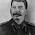Finally a simple way to illlustrate the dot and cross product. No more "multiplication of vectors" (which they aren't, by the way.) Maybe if more people used your methods, people would stop thinking physics is a bunch of random, esoteric, memorization problems.

5.nice one on the cross product :). along the same lines as one would go onto define a wedge product in the context of differential forms... very motivating

6.It is taking forever for your page to load. Perhaps not generating images dynamically and exporting the images as static PNGs would speed up your site, as it is pretty difficult to understand the explanation without appropriate images.

7.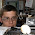Anonymous - Thanks for your comment. It seems that CodeCogs (who the equation images go through) is having some difficulties. I'm going to see if I can come up with a better way to do this.

8.i am just anable to understand when i will have to deal with cross product and when with dot product??????

9.Anonymous,
Put most simply: You use a dot product if you need to find bits of vectors that are parallel, you use a cross product if you need to find bits of vectors that are perpendicular.
Does that help?

10.Hello eli,

What does it mean by bits of vectors? Sort of like a component to it?

Could you give me some example of any physical meaning to dot and cross product?

11.Anonymous,
Yes, by bits'' I sort of mean components.

I'm not sure what you mean by physical meaning.'' I thought that's what I explained in this article? Do you want a physical application?

12.Sorry Eli. Yes, physical applications to it. Thanks Eli.

13.Anonymous,
Sorry for the delay. A physical application of the dot product arises when calculating work. The work is the integral of the force which acts along the direction of motion times the displacement, i.e. F . dr
A physical application of the cross product is used calculating torques. The torque is the force acting perpendicular to an arm times the length of the arm, or r x F
Does this help?

14.@Tim Poston- I don't understand this part of your argument:

"it is easy to show by elementary plane geometry -- with no use of right angles or baseXheight -- that C(u+w,v) = C(u,v) + C(w,v)"

This bewilders me. Can you explain this?

15.It _is_ easy -- just translate some triangles around -- but hard with only text. How can I add a diagram?

16.This comment has been removed by the author.

17.You can upload an image at http://imgur.com/ and then link to it here.

As far as the original problem of finding and intuitive solution for the area of a parallelogram, I found a nice animation that made it clear:
http://data.artofproblemsolving.com/aops20/resources/gallery/Side9.swf

18.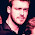Thanks dude!

I`m a young professor of math, and I managed to prepare a good discussion on the dot product, but until I found this blog, I could not do the same for the cross product.
Is always good to see that there are people who value discussions and real math over just methods and calculations with no context.

1.This comment has been removed by the author.

19.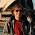But I have a problem with (9). Because the magnitude of A cross B is the area_3D and not A cross B.
I calculated it and i didn't get the area of the parallelogram...
Can you please explain me this equation?

1.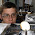Thanks for your comment. I am not entirely sure I understand your question, though. The magnitude of the cross product of A and B should be the (unsigned) area of the parallelogram. Can you give me a specific example where this does not work?

20.above interpretation of scaler and vector product is best.......but here is only one question in my mind that ABCOS(theta) and ABSin(theta) of dot and cross product respectively,both shows us magnitude but there is one additional step in the Dot one that we use unit vector to point out the direction of the vector quantity......so is it not possible that we get a vector quantity by taking both the vectors or their components in same direction i.e by dot product.........why is it neccessary that we will always have a vector quantity as a product of two vectors by taking Sin of theta between them or simply cross pproduct.......why the product (vector quantity)always depend on perpendicularity of two vectors.....why cant we get it from dot product....

21.above interpretation of scaler and vector product is best.......but here is only one question in my mind that ABCOS(theta) and ABSin(theta) of dot and cross product respectively,both shows us magnitude but there is one additional step in the cross one that we use unit vector to point out the direction of the vector quantity......so is it not possible that we get a vector quantity by taking both the vectors or their components in same direction i.e by dot product.........why is it neccessary that we will always have a vector quantity as a product of two vectors by taking Sin of theta between them or simply cross pproduct.......why the product (vector quantity)always depend on perpendicularity of two vectors.....why cant we get it from dot product....

1.I discuss this just after equation (3). In short, because the area has some directionality to it (there's a normal to a plane) which depends on which plane the area is in, we need a vector quantity for the area result.

22.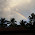With vector diagram prove c×(a+b)=c×a+c×b

23.Wow, I was trying to find the definition of the cross product using the dot product, something like:

A.V = 0
B.V = 0

Where A and B are some random nonparallel vector and V is a perpendicular vector from both.

This geometric proof is so simple and elegant.

Thank you for making this post.

1.Thanks for the positive feedback!